## Excel MONTH Function

If you want to extract the month number from a date format in Excel, the MONTH function can do you a favor. This tutorial is talking about the formula syntax and usage of MONTH function in Excel.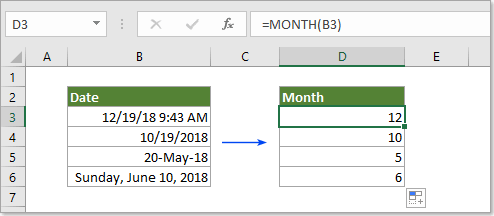Description of MONTH function

Syntax of MONTH function

Arguments of syntax

Example of MONTH function

#### Description of MONTH function

The Microsoft Excel MONTH function extracts the month from a date and displays as integer number from 1 to 12 (1 represents January and 12 represents December).

#### Syntax of MONTH function

=MONTH (serial_number)

#### Arguments of syntax

• Serial_number should be a valid date you will extract month from.

1) Directly reference to a cell containing the date: =MONTH(B3).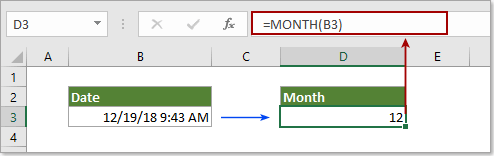2) Directly enter date as text string should be embraced with quotation marks: =MONTH("23-Aug-2012").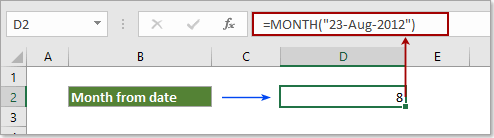3) Directly enter date as serial numbers: =MONTH(43424) (43424 represents date 11/20/2018).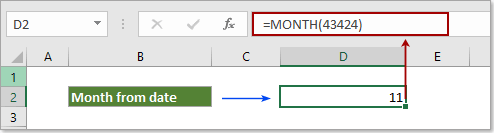4) Directly enter date as the result of a formula: =MONTH(DATE(2018,12,19)).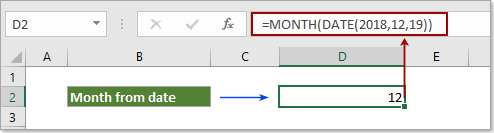#### Example of MONTH function

The below screenshot shows some examples of extracting months from dates.Results:

 Date Formula Description Result 12/19/18 9:43 AM =MONTH(A2) The serial_number is a valid Date/Time format 12 12/19/2018 =MONTH(A3) The serial_number contains no time element 12 // =MONTH(43424) The serial_number is a valid time format 11 // =MONTH(TODAY()) The serial_number is an invalid Date/Time format 12 10/25/2018 =CHOOSE(MONTH(A1),"Jan","Feb","Mar","Apr","May","June","July","Aug","Sept","Oct","Nov","Dec") The serial_number is corresponding decimal numbers Oct